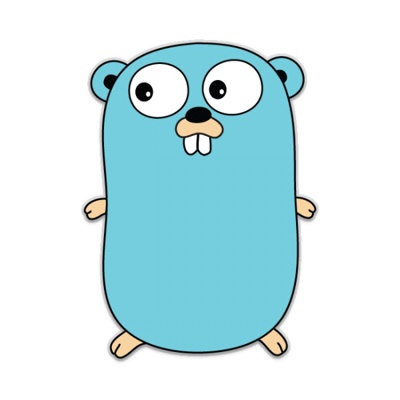# Interfaces in Golang

• 10 April 2020Interfaces are collections of method signatures. A interface type can hold any value that implements those methods.

#### Table of content

• Syntax
• Implementation
• The empty interface - is an interface type that specifies zero methods.
• Type assertion - A type assertion provides access to an interface underlying concrete value.
• Equality - Two interfaces are equal if they have equal concrete values and identical dynamic types, or if both are nil.
• Compile&Run - How to compile and run the Golang application

## Syntax

You can create an interface using the following syntax.

``````//interface_name interface description
type interface_name interface{

// Method signatures

}``````

For example we will take a simple interface that will define two methods.

``````//Animal the interface Animal defining two methods
type Animal interface {
Speak()
Move()
}``````

## Implement interface

A type implements an interface by implementing its methods. It does not contain any specific keyword to implement the interface, therefor it might be confusing. When you call a method on an interface value, the method of its underlying type is executed.

Let's take the classic example: We have two types `Cat` and `Pigeon`. If an interface defines method signatures for the methods `move` and `speak` and `Cat` and `Pigeon` implements these two methods (`move` and `speak`), then we can say that the types `Can` and `Pigeon` are implementing that interface.

### Example

We can have a look at the above example in practice.

``````package main

import "fmt"

//Animal the interface Animal defining two methods
type Animal interface {
Speak()
Move()
}

//Cat type
type Cat struct {
name string
}

//Pigeon type
type Pigeon struct {
name string
}

//Speak - implementing the Speak method for Cat
func (c Cat) Speak() {
fmt.Println(c.name, "says: meow meow.")
}

//Move implementing the Move method for Cat
func (c Cat) Move() {
fmt.Println(c.name, "can run.")
}

//Speak - implementing the Speak method for Pigeon
func (p Pigeon) Speak() {
fmt.Println(p.name, "says: coo coo.")
}

//Move implementing the Move method for Pigeon
func (p Pigeon) Move() {
fmt.Println(p.name, "can fly.")
}

func main() {
fmt.Println("Golang interface example")
fmt.Println()

cat := Cat{name: "Cat"}
cat.Move()
cat.Speak()

fmt.Println()

pigeon := Pigeon{name: "Pigeon"}
pigeon.Move()
pigeon.Speak()
}
``````

### Output

``````Golang interface example

Cat can run.
Cat says: meow meow.

Pigeon can fly.
Pigeon says: coo coo.``````

## The empty interface

The empty interface is an interface type that specifies zero methods. The syntax is:

``interface{}``

An empty interface may hold values of any type. The empty interfaces are useful in situations that handles values of unknown type.

### Example

``````package main

import "fmt"

func print(i interface{}) {
fmt.Printf("Value: %v, type: %T\n", i, i)
}

func main() {
fmt.Println("Empty interface example")
fmt.Println()

var empty interface{}

print(empty)

empty = 10
print(empty)

empty = 2.3
print(empty)

empty = "Hello world!"
print(empty)
}``````

### Output

``````Empty interface example

Value: <nil>, type: <nil>
Value: 10, type: int
Value: 2.3, type: float64
Value: Hello world!, type: string``````

## Type assertion

A type assertion provides access to an interface underlying concrete value. For example:

``str := val.(string)``

The example it will assert that the interface value of `val` holds a the concrete type of `string` and it will assign the underlying value of `val` to the variable `str`.

If `val` does not hold a `string`, the statement will trigger a panic.

To avoid this scenarion, the type assertion can return two values: the underlying value and a boolean value that reports if the assertion succeeded.

``str, ok := val.(string)``

If `ok` is `true`, then `str` it will take the underlying value. If `ok` is `false` the `str` it will take the zero value of the type (in this case `string`), and no panic will be triggered.

### Example

``````package main

import "fmt"

func main() {
fmt.Println("Golang type assertion example")
fmt.Println()

var val interface{} = "Hello World!!"

s := val.(string)
fmt.Println(s)

s, ok := val.(string)
fmt.Println(s, ok)

f, ok := val.(float64)
fmt.Println(f, ok)

//This will generate a panic
f = val.(float64)
fmt.Println(f)
}``````

### Output

``````Golang type assertion example

Hello World!!
Hello World!! true
0 false
panic: interface conversion: interface {} is string, not float64

goroutine 1 [running]:
main.main()
``````

## Equality

Two interface values are equal if one of the following statements are true:

• they have equal concrete values and identical dynamic types;
• both are nil;

### Example

Taking the previous example with `Cat` and `Pigeon` we can see in practice.

``````package main

import "fmt"

//Animal the interface Animal defining two methods
type Animal interface {
Speak()
Move()
}

//Cat type
type Cat struct {
name string
}

//Speak - implementing the Speak method for Cat
func (c Cat) Speak() {
fmt.Println(c.name, "says: meow meow.")
}

//Move implementing the Move method for Cat
func (c Cat) Move() {
fmt.Println(c.name, "can run.")
}

func main() {
fmt.Println("Golang interface equality example")
fmt.Println()

cat1 := Cat{name: "Cat"}
cat2 := Cat{name: "Cat"}

if cat1 == cat2 {
fmt.Println("The values are equals.")
}
}``````

### Output

``````Golang interface equality example

The values are equals.``````

## Compile&Run

To compile the code navigate to the file location and run the following command.

``\$ go build example.go``

Assuming that `example.go` is the name of your file.

Then depending on if you are on Linux or Windows the binary file is created.

To run the application, execute the command.

### Linux

``\$ ./example``

#### Windows

``c:\Users\adm\go\tutorials> example.exe``

If you want to compile and run the application in one single step, run the following command:

``go run example.go``